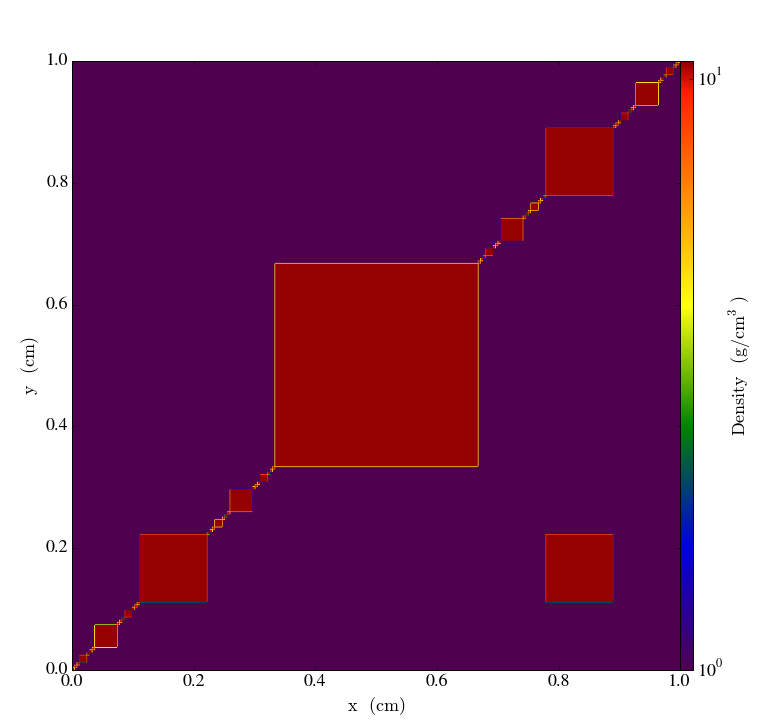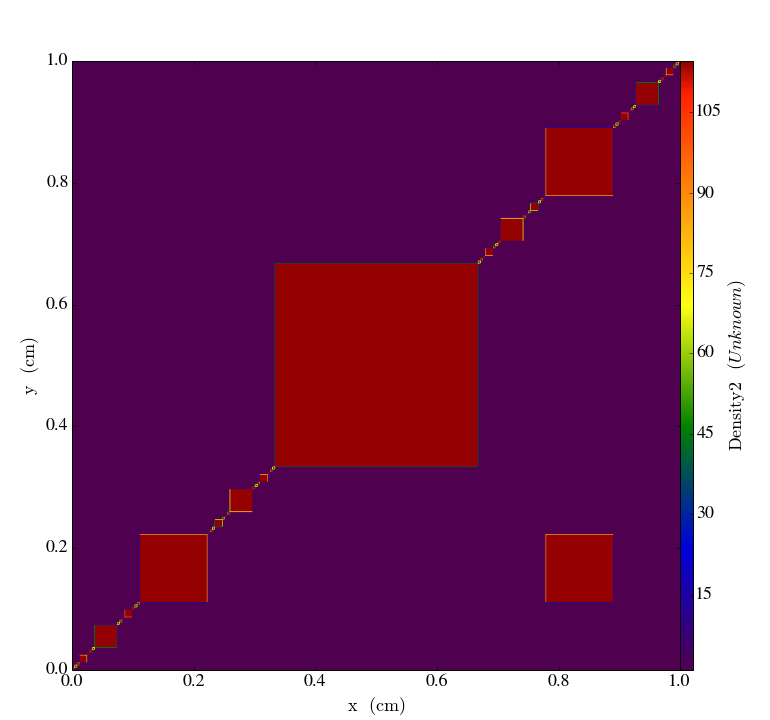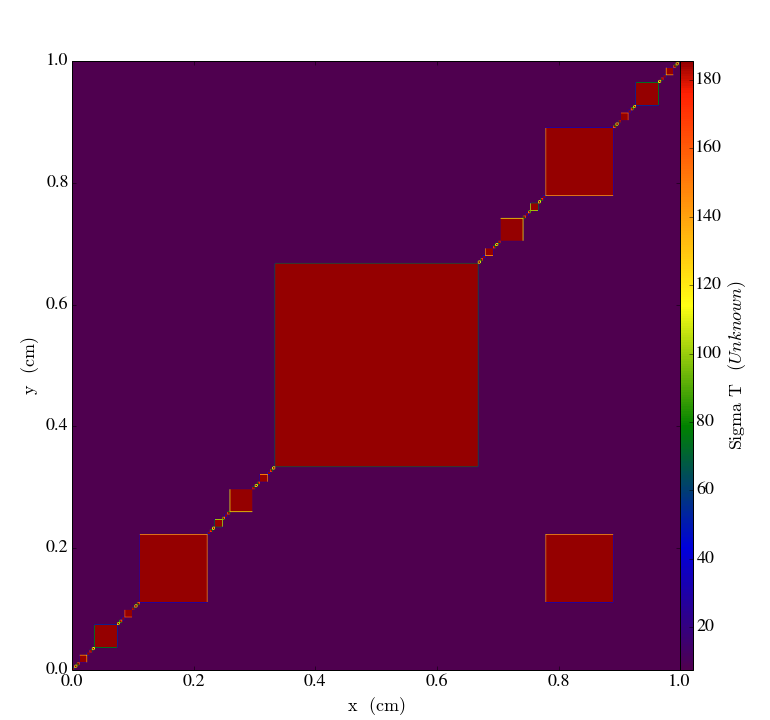# Mesh Tagging¶

Download the full notebook.

mesh_tags

# Let's Explore PyNE Meshes!¶

In :
from pyne.xs.channels import sigma_t
from pyne.material import Material, from_atom_frac
from pyne.mesh import Mesh, NativeMeshTag, MetadataTag, ComputedTag
from yt.config import ytcfg; ytcfg["yt","suppressStreamLogging"] = "True"
from yt.mods import *


First, let's create a 2D structured mesh, whose grid points in x & y are Cantor dust. Most of the mesh will have water as a coolant. Some volume elements will have fuel, though.

In :
def cantor(n):
return [0.] + cant(0., 1., n) + [1.]

def cant(x, y, n):
if n == 0:
return []
new_pts = [2.*x/3. + y/3., x/3. + 2.*y/3.]
return cant(x, new_pts, n-1) + new_pts + cant(new_pts, y, n-1)

In :
c5 = cantor(5)
coords = [c5, c5, [0.0, 1.0]]


We access volume elements (ve) on the mesh through a volume element index (idx). This index is a unique integer i that gives a sort ordering to an otherwise unorder mesh. The volume element index is defined on the range from 0 (inclusive) to the number of volumes in the mesh (exclusive). You may access a volume element's material through the mats attribute and indexing with i.

In :
m = Mesh(structured_coords=coords, structured=True)
fuel = from_atom_frac({'U235': 0.045, 'U238': 0.955, 'O16': 2.0}, density=10.7)
cool = from_atom_frac({'H1': 2.0, 'O16': 1.0}, density=1.0)
for i in range(len(m)):
m.mats[i] = cool
m.mats[len(m)/2] = fuel
m.mats[len(m)/4] = fuel
for i, c in enumerate(c5[:-1]):
m.mats[i*len(c5)] = fuel


## Tags¶

Tags - sometimes known as fields - are generic way of storing data on a mesh. Tags can be accessed as attributes on the mesh class itself. For example, the density tag may be grabbed via:

In :
m.density

Out:
MaterialPropertyTag(name='density', doc='the density [g/cc]')

To get the value of the density, you have to provide the location by its index.

In :
m.density

Out:
1.0

You can also slice, mask, or fancy index tags:

In :
print(m.density[::100])  # slice

[ 10.7   1.    1.    1.    1.    1.    1.    1.    1.    1.    1.    1.
1.    1.    1.    1.   10.7   1.    1.    1.    1.    1.    1.    1.
1.    1.    1.    1.    1.    1.    1.    1.   10.7   1.    1.    1.
1.    1.    1.    1. ]

In :
print(m.density[m.density[:] >= 10])  # mask

[ 10.7  10.7  10.7  10.7  10.7  10.7  10.7  10.7  10.7  10.7  10.7  10.7
10.7  10.7  10.7  10.7  10.7  10.7  10.7  10.7  10.7  10.7  10.7  10.7
10.7  10.7  10.7  10.7  10.7  10.7  10.7  10.7  10.7  10.7  10.7  10.7
10.7  10.7  10.7  10.7  10.7  10.7  10.7  10.7  10.7  10.7  10.7  10.7
10.7  10.7  10.7  10.7  10.7  10.7  10.7  10.7  10.7  10.7  10.7  10.7
10.7  10.7  10.7  10.7]

In :
print(m.density[[10, 0, 11, 100]])  # fancy index is fancy

[  1.   10.7   1.    1. ]


To get a list of all current tag names, uses the tags dictionary on the mesh:

In :
m.tags.keys()

Out:
['sub_act',
'sub_lan',
'number_density',
'density',
'comp',
'expand_elements',
'to_atom_frac',
'molecular_weight',
'mass_density',
'atoms_per_mol',
'sub_tru',
'mass',
'attrs',
'mult_by_mass',
'sub_ma',
'idx',
'sub_fp']

## Analysis & Visulaization¶

PyNE meshes are supported by the yt project. Use yt's plotting infrastructure to display tags on your mesh.

In :
pf = PyneMoabHex8StaticOutput(m)
s = SlicePlot(pf, 'z', 'density', origin='native')
s.display()## Computed Tags¶

Computed tags - also known as 'derived fields' - are a way of having a lazily evaluated 'virtual tag.' A computed tag is defined with a function, lambda, or any other callable object. The function must have the following interface:

def f(mesh, i):
"""mesh is a pyne.mesh.Mesh() object and i is the volume element index
to compute.
"""
# ... do some work ...
return anything_you_want


Here is a somewhat silly example which squares the density.

In :
m.density2 = ComputedTag(lambda mesh, i: mesh.density[i]**2)


Note that the bounds on the color bar have changed.

In :
pf = PyneMoabHex8StaticOutput(m)
s = SlicePlot(pf, 'z', 'density2', origin='native')
s.display()Here is a more serious example which uses PyNE's cross section tools to compute the one-group total cross section $\sigma_t$ everywhere on the mesh.

In :
m.sigma_t = ComputedTag(lambda mesh, i: sigma_t(mesh.mats[i], group_struct=[10.0, 1e-6], phi_g=[1.0]))

In :
pf = PyneMoabHex8StaticOutput(m)
s = SlicePlot(pf, 'z', 'sigma_t', origin='native')
s.display()Yes, that was only one line of code.